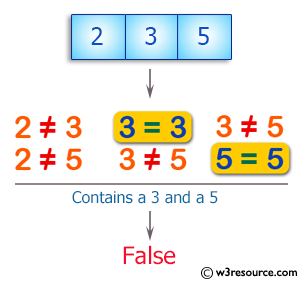﻿ Swift Array Programming Exercise: Test if an array of integers does not contain a 3 or a 5 - w3resource# Swift Array Programming Exercises: Test if an array of integers does not contain a 3 or a 5

## Swift Array Programming: Exercise-12 with Solution

Write a Swift program to test if an array of integers does not contain a 3 or a 5.

Pictorial Presentation:Sample Solution:

Swift Code:

``````func no35(_ a: [Int]) -> Bool {
if a.contains(3) || a.contains(5)
{
return false
}
else
{
return true
}
}
print(no35([2, 5]))
print(no35([2, 3, 5]))
print(no35([2, 4, 7]))
```
```

Sample Output:

```false
false
true
```

Swift Programming Code Editor:

Improve this sample solution and post your code through Disqus

What is the difficulty level of this exercise?

﻿